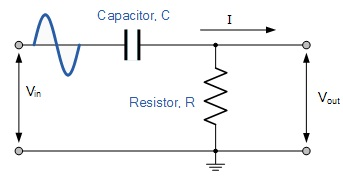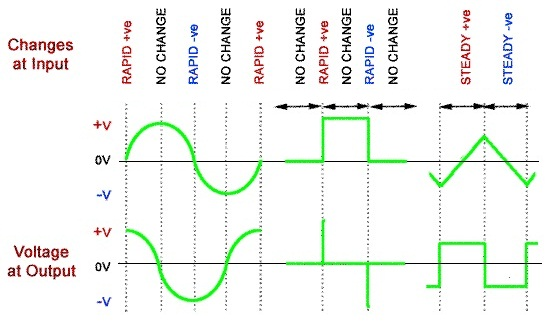RC Differentiator

Theory

A differentiator gives the derivative of input voltage as output. A differentiator using passive components resistors and capacitors is a high pass filter. The circuit is shown .It acts as a differentiator only when the time constant is too small. The voltage at output is proportional to the current through the capacitor. The current through the capacitor can be expressed as C dv / dt. The output is taking across the resistor. So output will be RC dv / dt. Thus differentiation of input takes place.

The circuit is called a differentiator because its effect is very similar to the mathematical function of differentiation, which means (mathematically) finding a value that depends on the rate of change of some quantity. The output wave of a differentiator circuit is ideally a graph of the rate of change of the voltage at its input. The output of a differentiator relates to the rate of change of its input, and that actually the actions of the high pass filter and the differentiator are the same.Fig.1 RC Differentiator circuit

The differentiator output is effectively a graph of the rate of change of the input. Whenever the input is changing rapidly, a large voltage is produced at the output. The polarity of the output voltage depends on whether the input is changing in a positive or a negative direction.A graph of the rate of change of a sine wave is another sine wave that has undergone a 90° phase shift (with the output wave leading the input wave). A square wave input produces a series of positive and negative spikes coinciding with the rising and falling edges of the input wave. A triangular wave has a steady positive going rate of change as the input voltage rises, so produces a steady positive voltage at the output. As the input voltage falls at a steady rate of change, a steady negative voltage appears at the output. The graph of the rate of change of a triangular wave is therefore a square wave. Although the ideal situation is shown here, how closely the output resembles perfect differentiation depends on the frequency (and periodic time) of the wave, and the time constant of the components used.